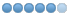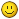# Servo Tuning PID and FFn

01 Nov 2011 12:07 #14483 by BigJohnT
This was snatched from another post and Jon did a good job of explaining FF0, FF1, and FF2. Now I need something in plain speak about PID.

Each of the FFs take some parameter from the commanded position and add a
value to the PID output. So, FF0 does commanded position * FF0 = value and then
adds that value to the pid output. FF1 is first derivative of position * FF1 = value
and FF2 is second derivative of position * FF2 = value.

So, when I say FF0 would be bad for an axis, it would cause a following error
that would be proportional to position. You could get the following error to zero
at one spot like X=1.2345", but there would be an accumulating error either side of that magic
location. This would not be a good thing.

So, FF0 is proportional to position (assuming an axis) or otherwise whatever parameter
is the input to the PID. FF1 is the first derivative of position, so that is proportional
to velocity. FF2 is second derivative of position, so it is proportional to acceleration.

I have not used the PID for a spindle application, but I can see that the PID is
given a position command for an axis, but a speed (velocity) for a spindle, so
there FF0 would be the right thing to adjust to compensate for finite servo
gain.

Jon

John

01 Nov 2011 14:14 - 01 Nov 2011 14:23 #14487 by andypugh
A PID controller performs some very simple calculations on the commanded value and the measured value, to produce a control output.

The P (for "Proportional") term is the simplest. it calculates the difference between the commanded value and the measured value, multiplies it by a fixed constant (the P-gain) and the result is the output.
Mathematically:
P = (Commanded - Measured) * Pgain.
The classic real-world example would be filling a bath to a fixed level. You would open the tap all the way while the difference between the actual level and the required level was large, and close it slowly until the bath level error was zero, at which point you would close it completely.

There are not many real-world examples where pure P-control works. Take the example of driving a car to the speed limit. You might press the pedalall the way to the floor to accelerate up to speed, but you don't apply zero throttle once you get up to speed, if you do that then you will slow down. If you stop driving now, and engage a very simple Cruise Control based only on a P-controller, then you would reach a speed just far enough below the required speed that the Pgain * speed error was enough to hold a steady speed. The higher the Pgain, the closer you get. Imagine that 25% pedal gives you 25 mph, and 50% pedal is 50mph. With a required speed of 50mph and a Pgain of 1, you would have 0% pedal at 50mph, 10% pedal at 40mph, and 25% pedal at 25mph, so your steady state speed would be only 25mph. With a Pgain of 100, then you would settle at 49.5mph. This sounds better, put what happens if you hit a gradient, and the speed falls to 49mph? The cruise control would immediately run to full throttle, then lift it all the way off at 50mph, then when the car slowed, run to full throttle again. P-controllers always do this, if you turn the Pgain too high, and this is why there is a limit to how high you can set the Pgain and have a stable loop. This also indicates that for many systems P-only control will never quite hit the setpoint. (Non-leaky baths are one of the exceptions)

To get that last bit of control, most controllers also have an I (for "Integral") term. This sounds a lot more technical than it is, and is normally described in textbooks in terms of calculus and greek symbols. But, if you look at any practical controller code, it is far more simple:
I(new) = I(old) + Igain * (Commanded - Measured).
So, every time the calculation is done, a fraction of the current error is added to the I term. Taking the example of the car above, with a P-gain of 1 the car sat at 25mph. If we add an I term with an Igain of 1, then the first time the loop runs, the I term will be 0 + 0.5 * 25mph = 12.5%, so the pedal position is now 37.5% (P + I), the next time the controller runs, the car is doing 37.5mph (this is an infinitely powerful massless car), so the I term is 12.5% + 6.25% = 18.75%. The P-term drops to 12.5% (because the error is less) and the pedal position is P + I = 31.25%, the car reaches 31.25mph...And so on.
Given enough time the car will reach a steady state very close to 50mph, with an I-term of 50% and a P term of zero.

What about that "D" term? Lets start with the car analogy. Imagine that you have your personal Pgain set rather high (and I think we have all met drivers like that). You want to accelerate to 50mph, and put your foot to the floor. The car turns out to be much higher-performance than you expected and you see that the speed is rising so quickly that you will over-shoot the speed limit, so you back off a bit. Despite the fact that you are still not even half-way to the speed you want. This is your own internal D (for "Differential") term. Again this is normally described in terms of calculus, but in the actual code it is something like.
D = Dgain * (New_measured - Old_Measured)
D gain is useful for avoiding overshoots, and can often allow you to run with a higher P-gain than would be stable otherwise. It can also help to slow the response to step-changes in command value (or, if required, enhance the response to step changes)

So, the final equation in the PID controller code is just P + I + D.
Last edit: 01 Nov 2011 14:23 by andypugh.
The following user(s) said Thank You: jo_key, snujcnc, SRDC, deanforbes, scubawarm, petmakris, LAUSCH

01 Nov 2011 15:21 #14488 by BigJohnT
Andy,

Thank you for that explanation... even I can comprehend that now. PID has always been a black box only privy to the select few who understood the Greek and higher math symbols used to describe it. It is rather clear to me now.

Thanks
John

• btvpimill
• Offline
• Elite Member
•02 Nov 2011 01:13 #14503
Andy, Simply Golden. You make it almost childs play.• jmelson
• Offline
• Moderator
•08 Nov 2011 20:09 #14696 by jmelson
Replied by jmelson on topic Re:Servo Tuning PID and FFn
btvpimill wrote:

Andy, Simply Golden. You make it almost childs play.Well, of course, it ISN'T child's play, because all these things interact, and all servos have finite
gain, and all systems have springiness and mass. So, this imposes additional effects that
come in in the form of natural vibrational frequencies. So, you can get resonances if
there is springiness and mass, and the servo loop adds energy at those frequencies.

But, Andy's explanation is a good foundation. The best thing is to set up a servo
system, learn how to use Halscope, and play with the servo response and how
the PID and FF1 & 2 affect it. Of course, the whole plan is to reduce following
error under a variety of situations to a minimum.

One of the bad features of the I term is that a machine tool axis is not a steady
state system, it is constantly accelerating and decelerating and reversing
direction, while cutting tools apply additional perturbations to the system.
A good I response while in one mode will adversely affect the system when the
load or direction changes. That's why I use it sparingly.

When you first try to set up a servo it will all seem magic, and the settings will
seem to have no rational connection to what happens. If you play with it for
a while, it will start to make sense.

Jon